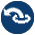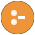#intercept.pl -- Intercept and signal interface

This library allows for creating an execution context (goal) which defines how calls to send_signal/1 are handled. This library is typically used to fetch values from the context or process results depending on the context.

For example, assume we parse a (large) file using a grammar (see phrase_from_file/3) that has some sort of record structure. What should we do with the recognised records? We can return them in a list, but if the input is large this is a huge overhead if the records are to be asserted or written to a file. Using this interface we can use

```document -->
record(Record),
!,
{ send_signal(record(Record)) },
document.
document -->
[].```

Given the above, we can assert all records into the database using the following query:

```    ...,
intercept(phrase_from_file(File, document),
record(Record),
assertz(Record)).```

Or, we can collect all records in a list using intercept_all/4:

```    ...,
intercept_all(Record,
phrase_from_file(File, document), record(Record),
Records).```
intercept(:Goal, ?Ball, :Handler)
Run Goal as call/1. If somewhere during the execution of Goal send_signal/1 is called with a Signal that unifies with Ball, run Handler and continue the execution.

This predicate is related to catch/3, but rather than aborting the execution of Goal and running Handler it continues the execution of Goal. This construct is also related to delimited continuations (see reset/3 and shift/1). It only covers one (common) use case for delimited continuations, but does so with a simpler interface, at lower overhead and without suffering from poor interaction with the cut.

Note that Ball and Handler are copied before calling the (copy) of Handler to avoid instantiation of Ball and/or Handler which can make a subsequent signal fail.

Compatibility
- Ciao
intercept(:Goal, ?Ball, :Handler, +Arg)
Similar to intercept/3, but the copy of Handler is called as `call(Copy,Arg)`, which allows passing large context arguments or arguments subject to unification or destructive assignment. For example:
```?- intercept(send_signal(x), X, Y=X).
true.

?- intercept(send_signal(x), X, =(X), Y).
Y = x.```
intercept_all(+Template, :Goal, ?Ball, -List)
True when List contains all instances of Template that have been sent using send_signal/1 where the argument unifies with Ball. Note that backtracking in Goal resets the List. For example, given
```enum(I, Max) :- I =< Max, !, send_signal(emit(I)),
I2 is I+1, enum(I2, Max).
enum(_, _).```

Consider the following queries

```?- intercept_all(I, enum(1,6), emit(I), List).
List = [1, 2, 3, 4, 5, 6].

?- intercept_all(I, (between(1,3,Max),enum(1,Max)),
emit(I), List).
Max = 1, List =  ;
Max = 2, List = [1, 2] ;
Max = 3, List = [1, 2, 3].```
- nb_intercept_all/4
nb_intercept_all(+Template, :Goal, ?Ball, -List)
As intercept_all/4, but backtracing inside Goal does not reset List. Consider this program and the subsequent queries
`enum_b(F, T) :- forall(between(F, T, I), send_signal(emit(I))).`
```?- intercept_all(I, enum_b(1, 6), emit(I), List).
List = [].

?- nb_intercept_all(I, enum_b(1, 6), emit(I), List).
List = [1, 2, 3, 4, 5, 6].```
send_signal(+Signal)
If this predicate is called from a sub-goal of intercept/3, execute the associated Handler of the intercept/3 environment.
Errors
- `unintercepted_signal(Signal)` if there is no matching intercept environment.
send_silent_signal(+Signal)
As send_signal/1, but succeed silently if there is no matching intercept environment.